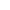# 钢板桩支护-淮安大运河深水基坑受力分析案例

2018-08-25

1725

1　工程概况

2   地质水文情况

3　围堰的计算依据及布置

3. 1　计算依据

3. 2　钢板桩布置

( kN /m3 )

°

kPa

① 耕土或素填土+ 6. 84 19. 4 0 0

② 青灰色、灰色亚砂土+ 6. 14 19. 5 19 7

③ 青灰色、灰黄色粘土+ 2. 74 19. 9 17 46

④ 灰黄色亚粘土+ 0. 34 19. 3 16 28

⑤ 黄色、黄灰色亚砂土- 0. 06 19. 8 24 9

⑥ 黄色粘土- 34. 9 20. 0 19 67

4　钢板桩围堰设计及计算

4. 1　土压力计算法分析4. 2各施工工况及内力计算

1)工况1　围堰第1道支撑( + 9. 68m)加好后,挖土、抽水到+ 3. 83m 标高时, 有效被动土压力h =5. 85m时, Pp’ = 124. 3kN /m2。2)工况2　围堰第2道支撑( + 4. 83m)加好后,挖土、抽水到+ 0. 03m 标高时, 有效被动土压力h =9. 65m时, Pp’ = 2 ×67 ×1. 402 = 187. 9 kN /m2。

σ =MmaxW= 73. 1 MPa < 0. 6 ×295 = 177 MPa,符合要求。

4. 3　钢板桩的最小入土深度

4. 4　坑底土抗隆起验算

4. 5　内支撑的设计计算

4. 5. 1工况1

1)可知圈梁最不利荷载出现在长边圈梁上, mmax

= 220.5kN·m, Nmax = 539. 4kN。则σ = 83. 5MPa≤f,强度符合要求。

2)长斜支撑钢管所受最大轴力N = 263. 2kN,计算长度l = 9. 7 m,由λ = l / i =52. 4,查表得:φ = 0. 877,则:σ =N / (φA) = 22. 9MPa≤f,整体稳定性符合要求。

3)短斜支撑钢管所受最大轴力N = 373. 8kN,计算长度l = 4. 1 m,由λ = l / i =22. 2,查表得:φ = 0. 977,图6　坑底隆起示意

4)直支撑钢管所受最大轴力N = 698. 1kN,计算长度l = 5 m,由λ = l / i = 27,查表得:φ = 0. 966,σ = N /(φA) = 55. 1 MPa≤f,整体稳定性符合要求。

4. 5. 2工况2

1)由计算可知,圈梁最不利荷载出现在长边圈梁上, mmax = 848. 1kN ·m, Nmax = 2 199. 6kN, 则, σ =164MPa≤f,强度符合要求。

2)长斜支撑钢管所受最大轴力N = 1 092. 3kN,计算长度l = 9. 7m,由λ = l / i =44. 4,查表得:φ = 0. 9,则:σ =N / (φA) = 52.1MPa≤f,整体稳定性符合要求。

3)短斜支撑钢管所受最大轴力N = 1 498. 9kN,计算长度l = 4. 1 m,由λ = l / i =18. 8,查表得:φ = 0. 983,则:σ =N / (φA) = 65.4MPa≤f,整体稳定性符合要求。

4)直支撑钢管所受最大轴力N = 2 835. 8kN,计算长度l = 5m,由λ = l / i = 22. 9,查表得:φ = 0. 975,则:σ = N / (φA ) = 124. 8 MPa≤f,整体稳定性符合要求。

5　结语

[ 1 ] 　地基处理手册[M ]. 北京:中国建筑工业出版社,1988.

[ 2 ] 　深基坑支护工程实例[M ]. 北京:中国建筑工业出版社,1996.